### Home > PC3 > Chapter 8 > Lesson 8.3.3 > Problem8-128

8-128.
1. Write each of the following expressions using sigma notation. Homework Help ✎

1.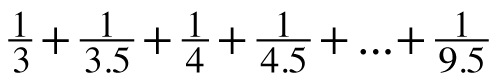2.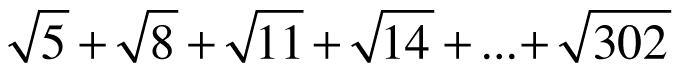3. cos(2) + 2 cos(4) + 3 cos(6) + … + 15 cos(30)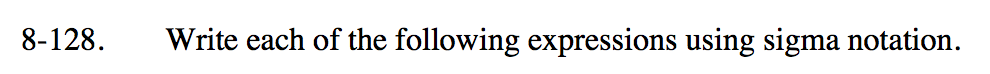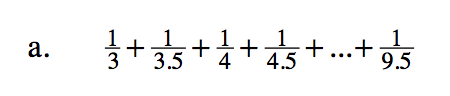$\displaystyle\sum\limits_{\textit{k}=0}^?\frac{1}{3+(?)\textit{k}}$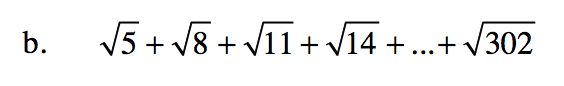$\displaystyle\sum\limits_{\textit{k}=0}^?\sqrt{5+(?)\textit{k}}$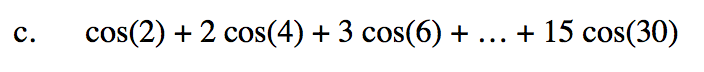Use the same thinking process as (a) and (b) above.